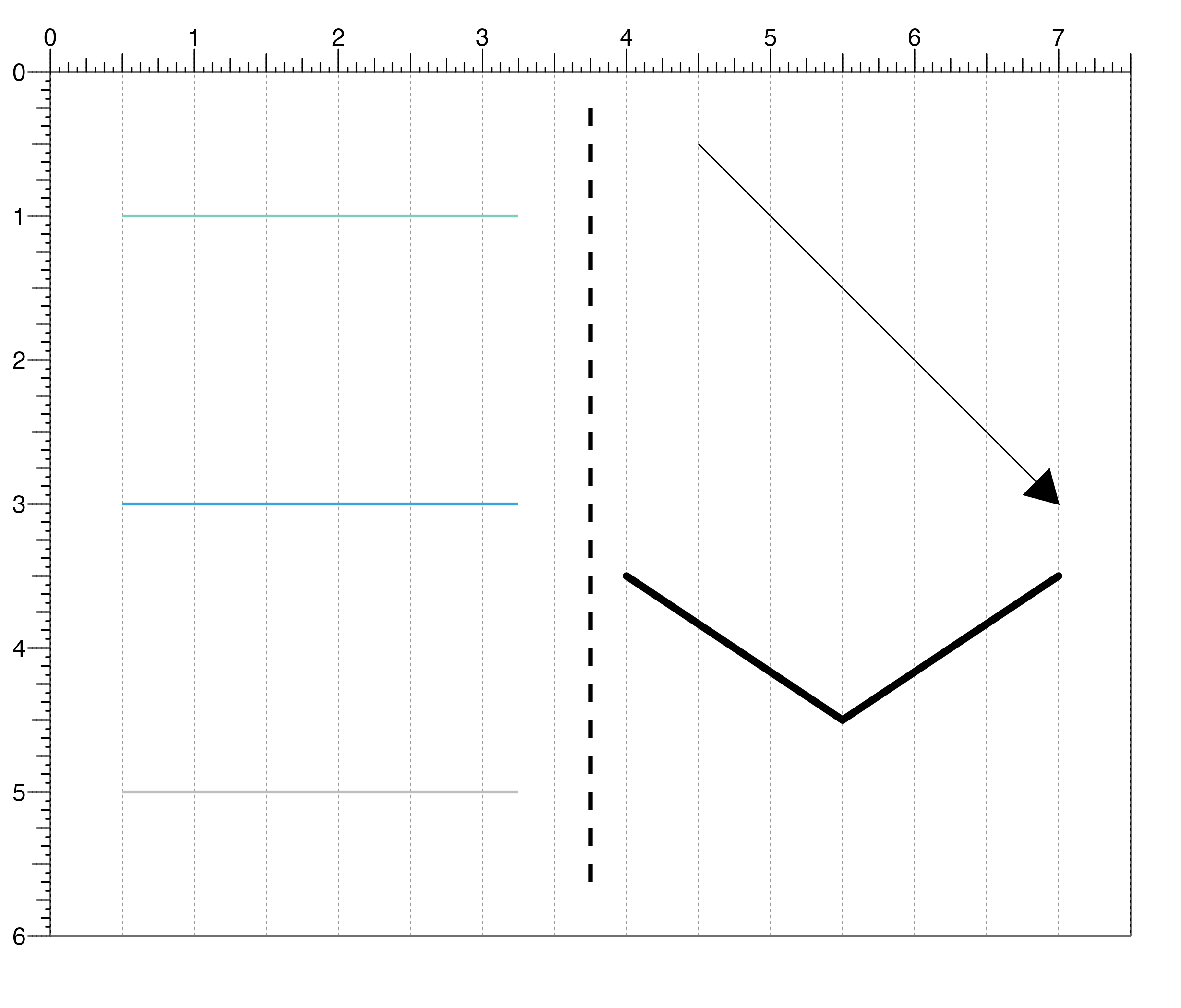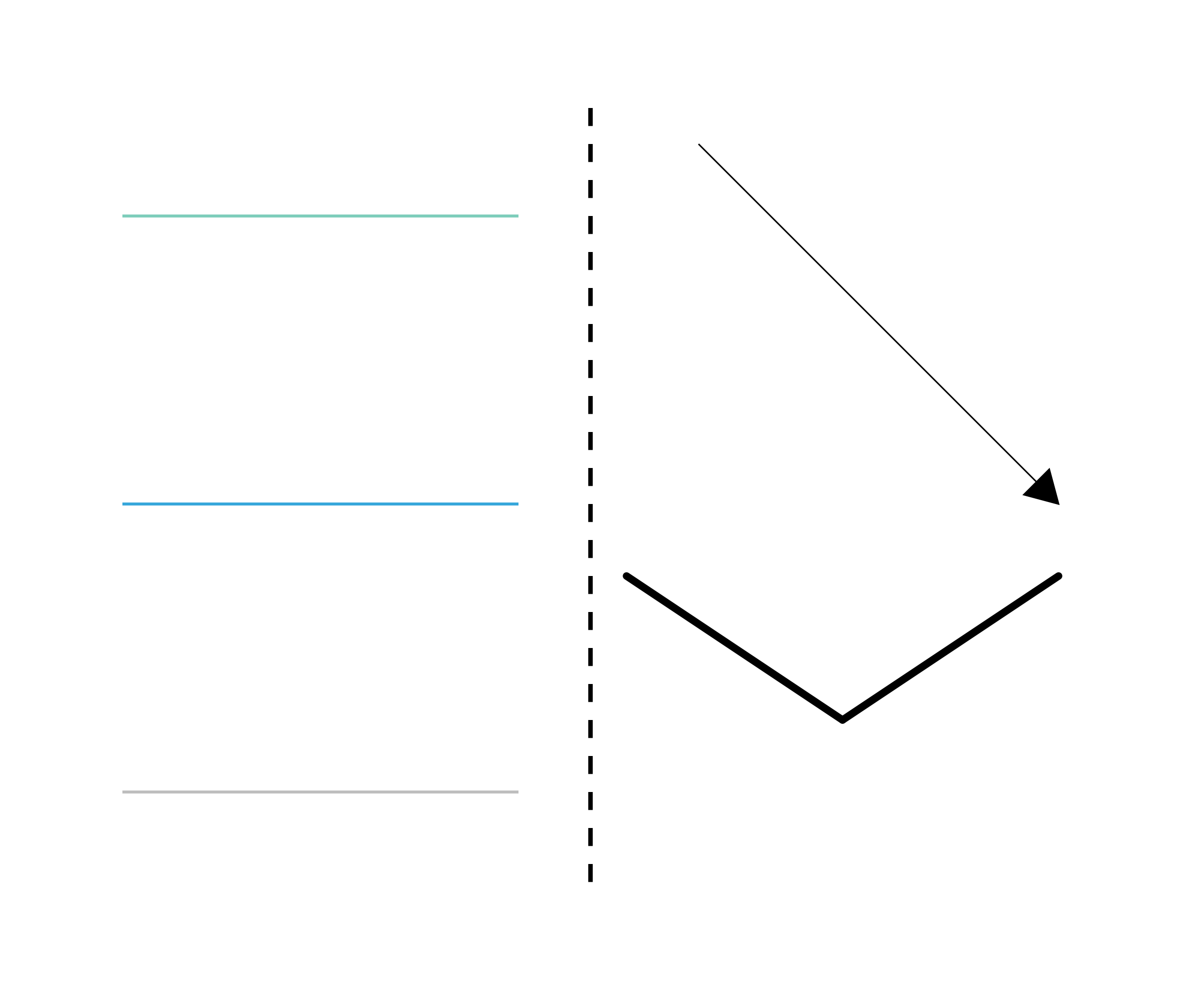Draw a line segment within a plotgardener layout

plotSegments(
x0,
y0,
x1,
y1,
default.units = "inches",
linecolor = "black",
lwd = 1,
lty = 1,
lineend = "butt",
linejoin = "mitre",
arrow = NULL,
params = NULL,
...
)

## Arguments

x0

A numeric vector or unit object indicating the starting x-values of the line segments.

y0

A numeric vector, unit object, or a character vector of values containing a "b" combined with a numeric value specifying starting y-values of the line segments. The character vector will place starting y-values relative to the bottom of the most recently plotted plot according to the units of the plotgardener page.

x1

A numeric vector or unit object indicating the stopping x-values of the line segments.

y1

A numeric vector, unit object, or a character vector of v alues containing a "b" combined with a numeric value specifying stopping y-values of the line segments. The character vector will place stopping y-values relative to the bottom of the most recently plotted plot according to the units of the plotgardener page.

default.units

A string indicating the default units to use if x0, y0, x1, or y1 are only given as numeric vectors. Default value is default.units = "inches".

linecolor

A character value specifying segment line color. Default value is linecolor = "black".

lwd

A numeric specifying segment line width. Default value is lwd = 1.

lty

A numeric specifying segment line type. Default value is lty = 1.

lineend

A character value specifying line end style. Default value is lineend = "butt". Options are:

• "round": Segment ends are rounded.

• "butt": Segment ends end exactly where ended.

• "square": Segment ends are squared.

linejoin

A character value specifying line join style. Default value is linejoin = "mitre". Options are:

• "round": Line joins are rounded.

• "mitre": Line joins are sharp corners.

• "bevel": Line joins are flattened corners.

arrow

A list describing arrow heads to place at either end of the line segments, as produced by the arrow function.

params

An optional pgParams object containing relevant function parameters.

...

Additional grid graphical parameters. See gpar.

## Value

Returns a segments object containing relevant placement and grob information.

## Examples

library(grid)
## Create a page
pageCreate(width = 7.5, height = 6, default.units = "inches")

## Plot one line segment
plotSegments(
x0 = 3.75, y0 = 0.25, x1 = 3.75, y1 = 5.75,
default.units = "inches",
lwd = 3, lty = 2
)
#> segments[segments1]

## Plot multiple line segments at different locations in different colors
plotSegments(
x0 = 0.5, y0 = c(1, 3, 5), x1 = 3.25, y1 = c(1, 3, 5),
default.units = "inches",
lwd = 2, linecolor = c("#7ecdbb", "#37a7db", "grey")
)
#> segments[segments2]

## Plot a line segment with an arrowhead
plotSegments(
x0 = 4.5, y0 = 0.5, x1 = 7, y1 = 3,
default.units = "inches",
arrow = arrow(type = "closed"), fill = "black"
)
#> segments[segments2]

## Plot lines with round lineends
plotSegments(
x0 = c(4, 7), y0 = 3.5, x1 = 5.5, y1 = 4.5,
default.units = "inches",
lwd = 5, lineend = "round"
)
#> segments[segments2]## Hide page guides
pageGuideHide()